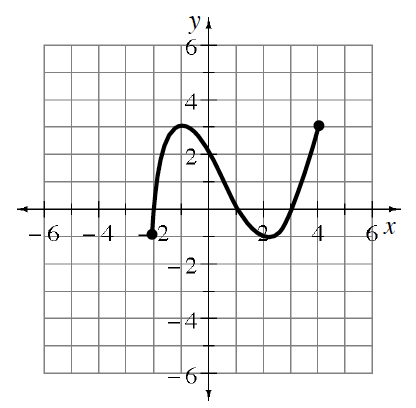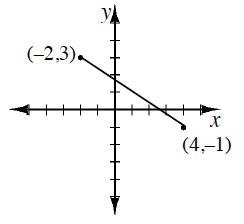### Home > INT3 > Chapter 1 > Lesson 1.1.3 > Problem1-35

1-35.

Examine the function $y=g(x)$ graphed at right.1. Which $x$-values have points on the graph? That is, describe the domain of $g$.

The domain includes $-2, 4$ and all the numbers between. $-2 ≤ x ≤ 4$.

2. What are the possible outputs for $g(x)$? That is, what is the range?

The range includes $-1,3$, and all the numbers between. $-1 ≤ y ≤ 3$.

3. Ricky thinks the range of $g(x)$ is $–1, 0, 1, 2,$ and $3$. Is he correct? Why or why not?

Is there a point on the graph for $x = 2.5?$ or for $x = -0.33?$

He is not correct since he is missing all the values between his numbers.
The curve is continuous, so the description needs to include all numbers, not just integers.

4. Make a sketch of another function with the same domain and range as $g$.

There are many possibilities. Here is one example.